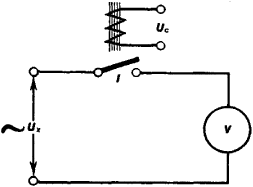# Vectormeter

The following article is from The Great Soviet Encyclopedia (1979). It might be outdated or ideologically biased.

## Vectormeter

an electrical instrument for the measurement of the average values of the intensity and phase of alternating current or a voltage. When the quantity measured has no even harmonics, a vectormeter makes it possible to measure the instantaneous current and voltage magnitudes and to plot a curve of their variation with time. A circuit that explains the principle of the instrument is shown in Figure 1 : The AC voltage Ux being studied is fed to the terminals of a magnetic-electric voltmeter V through an interrupter I that is operated by an electromagnet connected to an auxiliary control voltage Uc . When the phase of voltage Ux coincides with that of Uc , the contacts of I are closed and remain in this position during the positive half-period of the variation in Ux ; in this case the voltmeter indicates half the average value of the voltage Ux . When the phase of voltage Ux changes with respect to the phase of voltage Uc , the voltmeter will receive during some portion of the period negative voltage of the second half-period, and the instrument’s reading will decrease. With a phase shift of 90° between Uc and Ux the voltmeter will read zero. The source of the control voltage is equipped with an instrument (with a scale) for reading the phase of Uc . By varying the phase of Uc to obtain the maximum voltmeter reading—that is, to the point of coincidence of phase of the voltages Uc and Ux —the phase of Ux is found from the scale on the source of the control voltage. Soviet industry produces a vectormeter of this type that uses a micromotor as the interrupter I . These instruments, which are designed for measuring AC circuits with a frequency of 50 hertz, have a range of voltage measurements from 0.15 to 300, current measurements from 0.003 to 5 amperes, and phase measurements from 0° to 360°. The measuring ranges can be changed by the supplementary connection of external shunts or discrete auxiliary resistors and instrument transformers. Vectormeters are used for laboratory investigations of complex electrical circuits and devices and for testing the magnetic properties of electrical steels.Figure 1. Functional diagram of a vectormeter

N. G. VOSTROKNUTOV